Link to YouTube playlist for videos that accompany each chapter

# Chapter 8 ggplot2: Data Visualization Using The Grammar of Graphics

Figure 8.1: How many pattern violations do you see?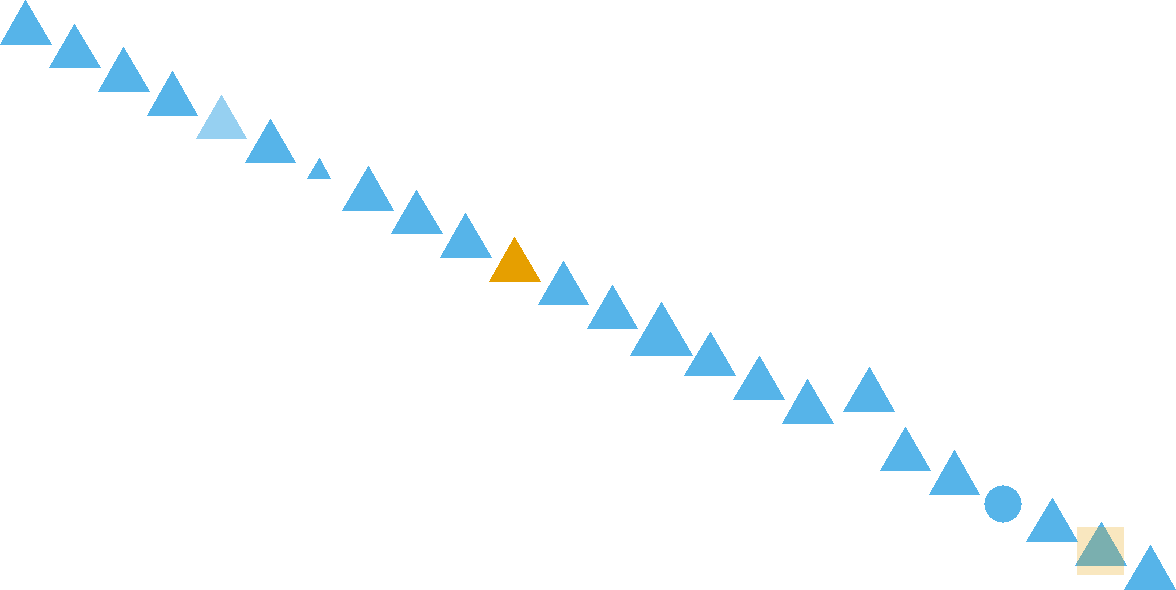A large portion of thoughts and concepts in this chapter are inspired by Noah Ilinsky. See his talk here: (https://youtu.be/R-oiKt7bUU8)

## 8.1 Why Visualize Data?

Visualization makes data accessible to the human brain. Evolution has wired our eyes and brain to be very sophisticated in pattern recognition. This includes detecting patterns and violations of patterns in regards to position, color, size, shape, gaps, trends, etc. For example, in Figure 8.1, your brain will easily detect seven pattern violations.

To take advantage of the facile nature with which our eyes digest visual information, we will learn to map data - like a column of a data frame - to properties of visual markings made on the screen or on paper. These properties, called aesthetics, include things like position, shape, color, and transparency. By intelligently mapping data to aesthetics, we can not only see our data, but lead ourselves and our audience to visual insight.

### 8.1.1 Anscombe’s Quartet: A Case For Visual Revelation

Data presented in tables or even in statistical summaries are rarely as forthcoming with insight as is a good visualization. Anscombe (1973)Anscombe, F. J. 1973. “Graphs in Statistical Analysis.” The American Statistician 27 (1): 17–21. constructed four fictitious datasets to illustrate this point - each dataset consisting of x-y value pairs: {(x1,y1),(x2,y2),(x3,y3),(x4,y4)}. The four datasets, known as Anscombe’s quartet, have virtually indiscernible statistical properties. However, the distinguishing characteristics of each dataset are very evident when graphed. Due to the cogency of the arguments made by Anscombe, R programmers include the anscombe dataset as aprt of the R installation. We can see the data in tabulated form using the following lines:

library("dplyr")

## retrieve the anscombe dataset
ansDF = anscombe %>% as_tibble()

# notice the x-values for the first three datasets
# are the same and scanning the y-values yields
# little insight
ansDF
## # A tibble: 11 x 8
##       x1    x2    x3    x4    y1    y2    y3    y4
##    <dbl> <dbl> <dbl> <dbl> <dbl> <dbl> <dbl> <dbl>
##  1    10    10    10     8  8.04  9.14  7.46  6.58
##  2     8     8     8     8  6.95  8.14  6.77  5.76
##  3    13    13    13     8  7.58  8.74 12.7   7.71
##  4     9     9     9     8  8.81  8.77  7.11  8.84
##  5    11    11    11     8  8.33  9.26  7.81  8.47
##  6    14    14    14     8  9.96  8.1   8.84  7.04
##  7     6     6     6     8  7.24  6.13  6.08  5.25
##  8     4     4     4    19  4.26  3.1   5.39 12.5
##  9    12    12    12     8 10.8   9.13  8.15  5.56
## 10     7     7     7     8  4.82  7.26  6.42  7.91
## 11     5     5     5     8  5.68  4.74  5.73  6.89

Notice how the x-values for each of the first three datasets, (i.e. x1, x2, and x3) are the same, yet patterns in the corresponding y-values (i.e. y1, y2, and y3, respectively) are not easily discernible. This tabulated form of data yields little insight.

One might think that statistical transformations can be used to yield more insight. So let’s try this using a statistical transformation of the data that summarize’s the relationship between respective x’s and y’s using the equation of a line, i.e. a linear regression. The lm function in R can be used to get linear regression output. For this book, basic linear regression knowledge is assumed - for an introduction or refresher on linear regression, please consult OpenIntro’s introductory statistics textbook: https://www.openintro.org/book/os/ Notice (below) that the output for both the slope coefficient ($$\approx 0.5$$) and the y-intercept ($$\approx 3$$) is nearly identical for all four datasets and one might (wrongly) assume the datasets to be quite similar as a result:

model1 = lm(y1 ~ x1, data = ansDF)  ##predict y1 using x1
model2 = lm(y2 ~ x2, data = ansDF)  ##predict y2 using x2
model3 = lm(y3 ~ x3, data = ansDF)  ##predict y3 using x3
model4 = lm(y4 ~ x4, data = ansDF)  ##predict y4 using x4
##show results of regression (i.e. intercept and slope)
coef(model1)
## (Intercept)          x1
##   3.0000909   0.5000909
coef(model2)
## (Intercept)          x2
##    3.000909    0.500000
coef(model3)
## (Intercept)          x3
##   3.0024545   0.4997273
coef(model4)
## (Intercept)          x4
##   3.0017273   0.4999091

Despite the statistical transformation (i.e. regression output) yielding nearly identical insights, visualizing the data, as shown in Figure 8.2, tells a much richer story; the type of story we want to tell as we use data visualization for both exploratory analysis and managerial persuasion.

Figure 8.2: This visual depiction of Ansombe’s quartet yields much more insight than simply viewing the data in tabular form or relying on output of a linear regression.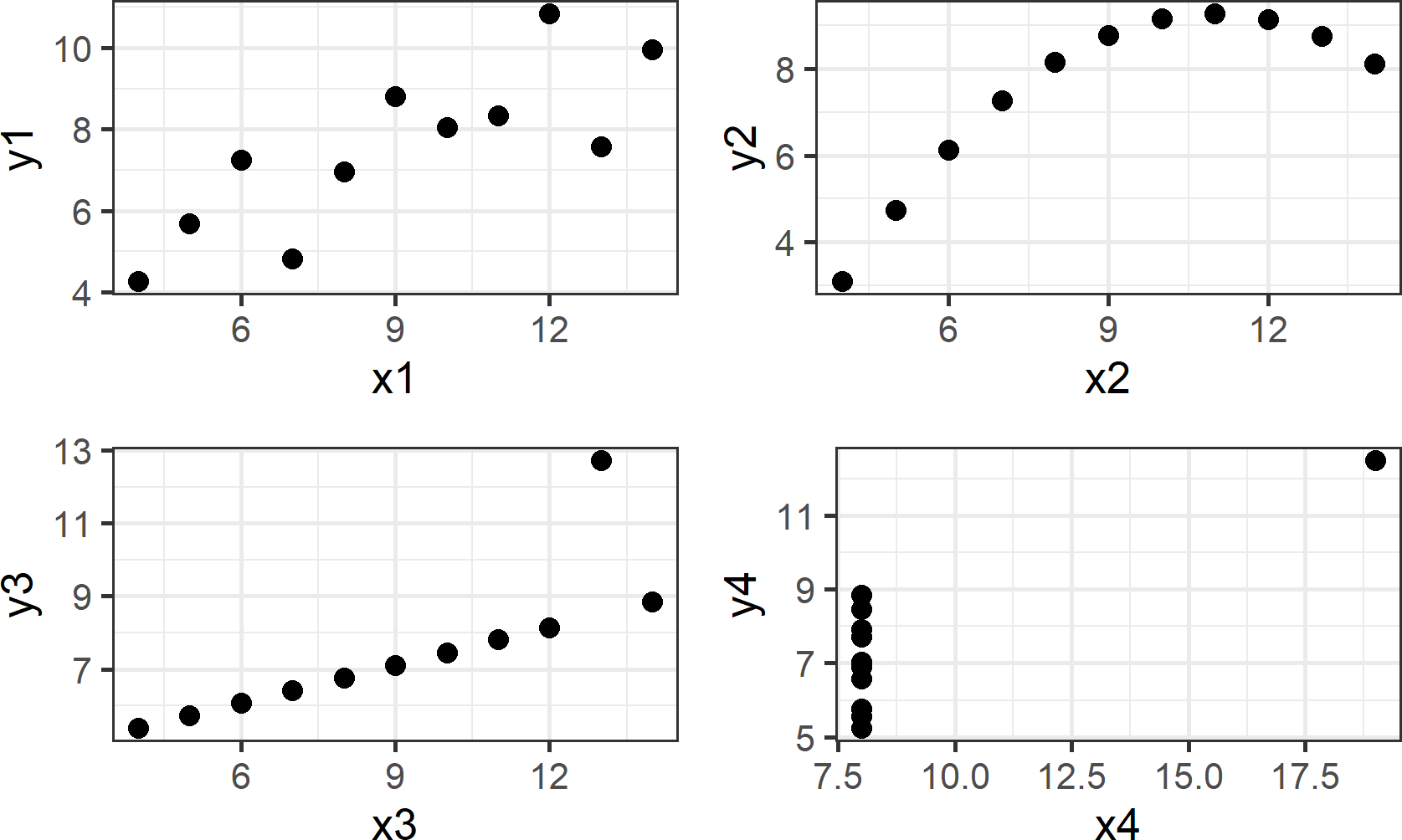Going forward, I will ask you to change the way you look at plots such as those shown above. Specifically, I request that you think of plots as representing a mapping of data to visual properties which you see on a screen or paper. To this end, take notice of the visual markings in each of the four plots. For each plot, one X column and one Y column is extracted - these are your data. For example,

ansDF %>% select(x2,y2)
## # A tibble: 11 x 2
##       x2    y2
##    <dbl> <dbl>
##  1    10  9.14
##  2     8  8.14
##  3    13  8.74
##  4     9  8.77
##  5    11  9.26
##  6    14  8.1
##  7     6  6.13
##  8     4  3.1
##  9    12  9.13
## 10     7  7.26
## 11     5  4.74

yields 11 rows (or observations) of $$(x,y)$$ pairs for the upper-right plot of Figure 8.2. For each observation, the $$x$$-value is mapped to horizontal position and the $$y$$-value is mapped to vertical position: \begin{aligned} \textrm{x} &\rightarrow \textrm{horizontal postion}\\ \textrm{y} &\rightarrow \textrm{vertical postion}\\ \end{aligned} To display the mapped visual aesthetics, a geom or visual marker is used - in our example this geom was chosen to be points: $\textrm{geom} = \textrm{points}$ An alternative aesthetic mapping and geom selection would be the following:

\begin{aligned} x &\rightarrow \textrm{horizontal position}\\ y &\rightarrow \textrm{fill color}\\ \textrm{geom} &= \textrm{rectangular bar} \end{aligned}

Figure 8.3: Plot showing an alternative mapping of data to aesthetics and alternative geom. Notice this plot is less effective at revealing the pattern seen in the previous plot.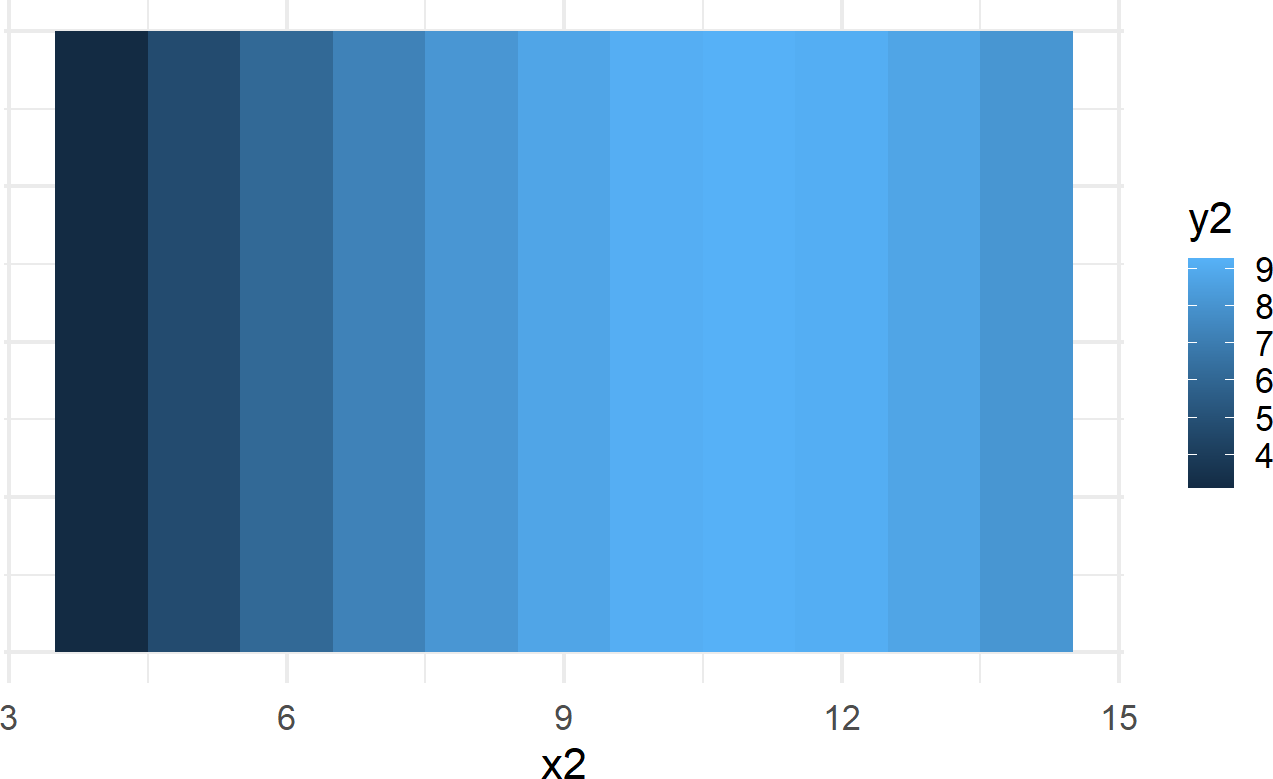While Figure 8.2 (upper right-hand plot) is far superior in revealing the curvilinear relationship between $$x2$$ and $$y2$$ than Figure 8.3, they both are representations of the exact same data. For example, notice the maximum $$y2$$ value of Figure 8.2 is now represented by the lightest shading in Figure 8.3; both are viusal representation of the exact same data point $$(x2,y2) = (11,9.26)$$ with the first representation being the more usful of the two.

The key lessons to takeaway from this exploration of Anscombe’s quartet are the following:

• Tabulated data is a struggle to read and obscures discovery of patterns or relationships.
• Statistical transformations tell stories, but the true story of the underlying data might be missed if not visualized.
• Graphing data enables us to readily see patterns that tabulation or statistical tests fail to help us with.
• Mapping of data to aesthetic properties and visualization using different geoms impact the effectiveness of a visualization’s ability to reveal underlying patterns in the data.

## 8.2 ggplot2: Using the Grammar of Graphics

In this section, we show how to specify the mapping of data to aesthetic properties and visual markings using the ggplot2 package (Wickham 2009Wickham, Hadley. 2009. Ggplot2 Elegant Graphics for Data Analysis. Springer-Verlag New York. http://ggplot2.org.) that is part of the tidyverse package group (Wickham 2017Wickham, Hadley. 2017. Tidyverse: Easily Install and Load the ’Tidyverse’. https://CRAN.R-project.org/package=tidyverse.). The mapping is accomplished via a set of rules known as the grammar of graphics.

In language, rules of grammar are used to convey meaning when words are combined. For example, Figure 8.4 is similar to a meme circulating on Facebook that shows how English grammar, in this case spacing and the use of a hyphen, changes the meaning of words.

Figure 8.4: Grammar helps to convey meaning efficiently. Humorously, this cartoon compares the grammatical implications of spacing and hyphens. A one-night stand is suggestive of a short romantic encounter whereas a nightstand is simply a bedside table.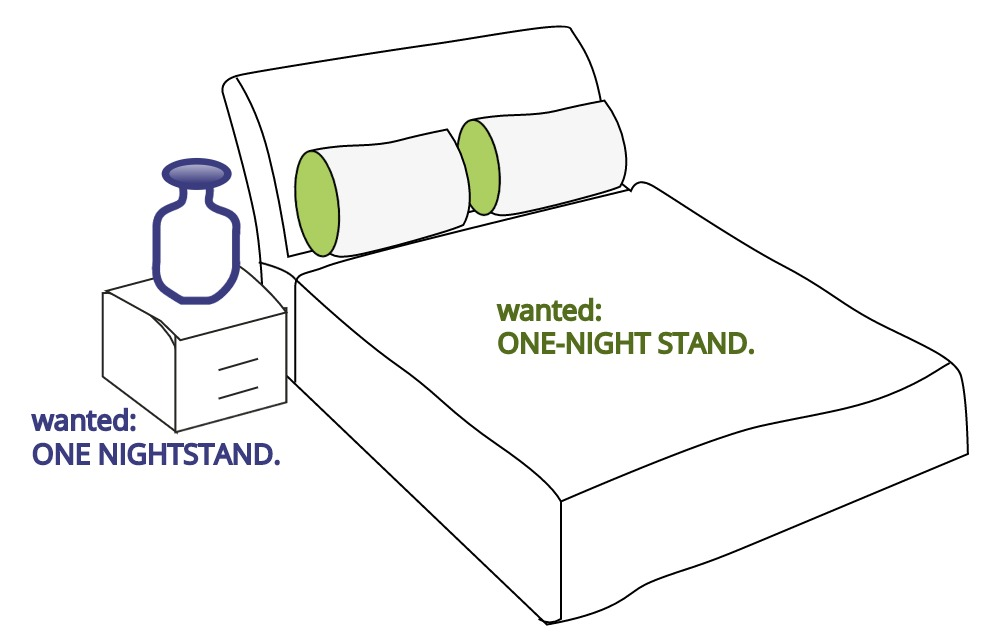Through these rules, readers can correctly comprehend the meaning an author wishes to convey. Just like with words, graphics also have an underlying grammar which can be leveraged to accurately describe a graphic or visual. This grammar, formalized in the lengthy and terse work of Wilkinson (2006)Wilkinson, Leland. 2006. The Grammar of Graphics. Springer Science & Business Media. has thankfully been made much more accessible to R-users via Hadley Wickham’s excellent ggplot2 package. Once we learn to use this grammar properly, good graphics become easier to both describe and create.

### 8.2.1 Specifying a Plot

English grammatical rules specify that a complete sentence satisfies three conditions:

1. It begins with a capital letter.
2. It includes an ending punctuation mark like a period(.) or question mark(?).
3. It contains a main clause with a subject and verb.

Analogously, there are conditions required by the ggplot2 package’s implementation of the grammar of graphics to specify a complete plot:

1. It begins with a dataset.
2. It includes a geometric object, called a geom, along with that geom’s minimal required set of aesthetic mappings which specify how data is to be transformed into a visual display.

We can use the starwars dataset from the dplyr package to illustrate these two conditions. It can be accessed like any other data frame:

starwars
## # A tibble: 87 x 14
##    name    height  mass hair_color  skin_color eye_color birth_year sex   gender
##    <chr>    <int> <dbl> <chr>       <chr>      <chr>          <dbl> <chr> <chr>
##  1 Luke S~    172    77 blond       fair       blue            19   male  mascu~
##  2 C-3PO      167    75 <NA>        gold       yellow         112   none  mascu~
##  3 R2-D2       96    32 <NA>        white, bl~ red             33   none  mascu~
##  4 Darth ~    202   136 none        white      yellow          41.9 male  mascu~
##  5 Leia O~    150    49 brown       light      brown           19   fema~ femin~
##  6 Owen L~    178   120 brown, grey light      blue            52   male  mascu~
##  7 Beru W~    165    75 brown       light      blue            47   fema~ femin~
##  8 R5-D4       97    32 <NA>        white, red red             NA   none  mascu~
##  9 Biggs ~    183    84 black       light      brown           24   male  mascu~
## 10 Obi-Wa~    182    77 auburn, wh~ fair       blue-gray       57   male  mascu~
## # ... with 77 more rows, and 5 more variables: homeworld <chr>, species <chr>,
## #   films <list>, vehicles <list>, starships <list>

To create a visual, we pass this dataframe as the argument value for the data argument in the ggplot function call:

library("ggplot2") ##load for plotting

ggplot(data = starwars) +
geom_point(mapping = aes(x = height, y = mass))

Figure 8.5: Output from ggplot() function - note, your output might look slightly different due to some graphical defaults that I use behind the scenes.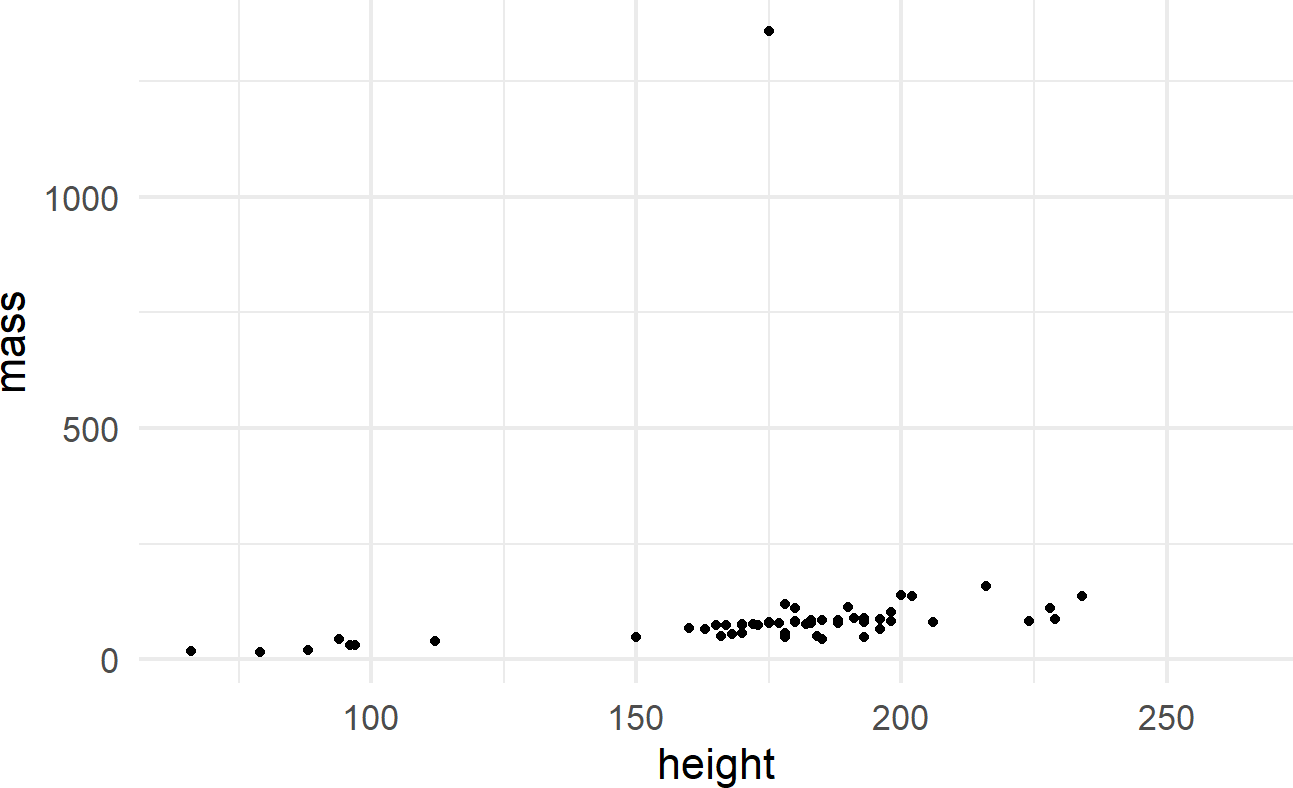# The "+" is used to add layers to a plot
# For ggplot, we use "+" not "%>%"

The initial ggplot() function call initiates the creation of a plot using a given dataset. The geom_point() function adds a layer of visual markings, i.e. point geoms, to the plot where:

• each point represents one row of the starwars dataframe,
• the x-position of each point is determined by the value of the height variable,
• the y-position of each point is determined by the value of the mass variable, and
• intelligent defaults are set for every other decision required to make the corresponding plot.

See https://ggplot2.tidyverse.org/reference/#section-layer-geoms for complete list of available geometric objects.

### 8.2.2 Simple Plot Variations

Add another aesthetic mapping for a useful variation. To discover more aesthetics that can be controlled when using geom_point(), use ?geom_point to open the Help pane in the lower-right of RStudio. Scrolling down, you will discover the aesthetics shown in Figure 8.6 can be controlled when using this layer.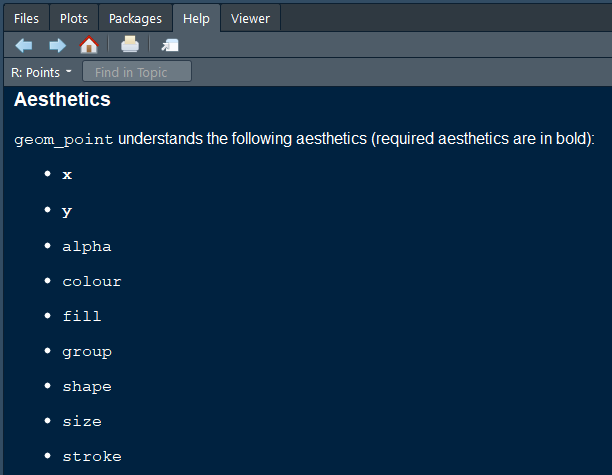Figure 8.6: Aesthetics that can be controlled when using geom_point().

One way to control an aesthetic is to map it to the data by specifying the mapping within the aes() function call.

ggplot(data = starwars) +
geom_point(mapping =
aes(x = height,
y = mass,
color = gender))The other way is to map the aesthetic to a constant outside of the aes() function, but within the geom function, like this:

ggplot(data = starwars) +
geom_point(mapping =
aes(x = height, y = mass),
shape = 15, color = "red")where color and shape are specified outside of the aes() function because they are mapped to constant values and not a column of the dataframe.

For more information on what shapes and colors are available, execute the following R function vignette("ggplot2-specs") to open up details in the Help pane of RStudio.

Multiple layers of geoms can be put on one plot. For example, we might want to name the points:

ggplot(data = starwars) +
geom_point(mapping = aes(x = height, y = mass)) +
geom_text(mapping =
aes(x = height, y = mass, label = name),
check_overlap = TRUE)To make the function call more concise, we can avoid the redundancy of mapping x-y positions for every geom by specifying mapping defaults in the initial ggplot() function. Any geom layers will use these defaults unless overridden with an aes() call from within that geom. Additionally, we can omit the data and mapping argument names by specifying those argument values in the order that the function expects. This yields the same plot with a little less typing:

Using check_overlap = TRUE omits data labels that would otherwise overwrite and obscure a previously drawn data label. Experiment leaving this argument out of the geom_text() function to see the ugliness that happens when every label is printed.

ggplot(starwars, aes(x = height, y = mass)) +
geom_point() +
geom_text(aes(label = name), check_overlap = TRUE)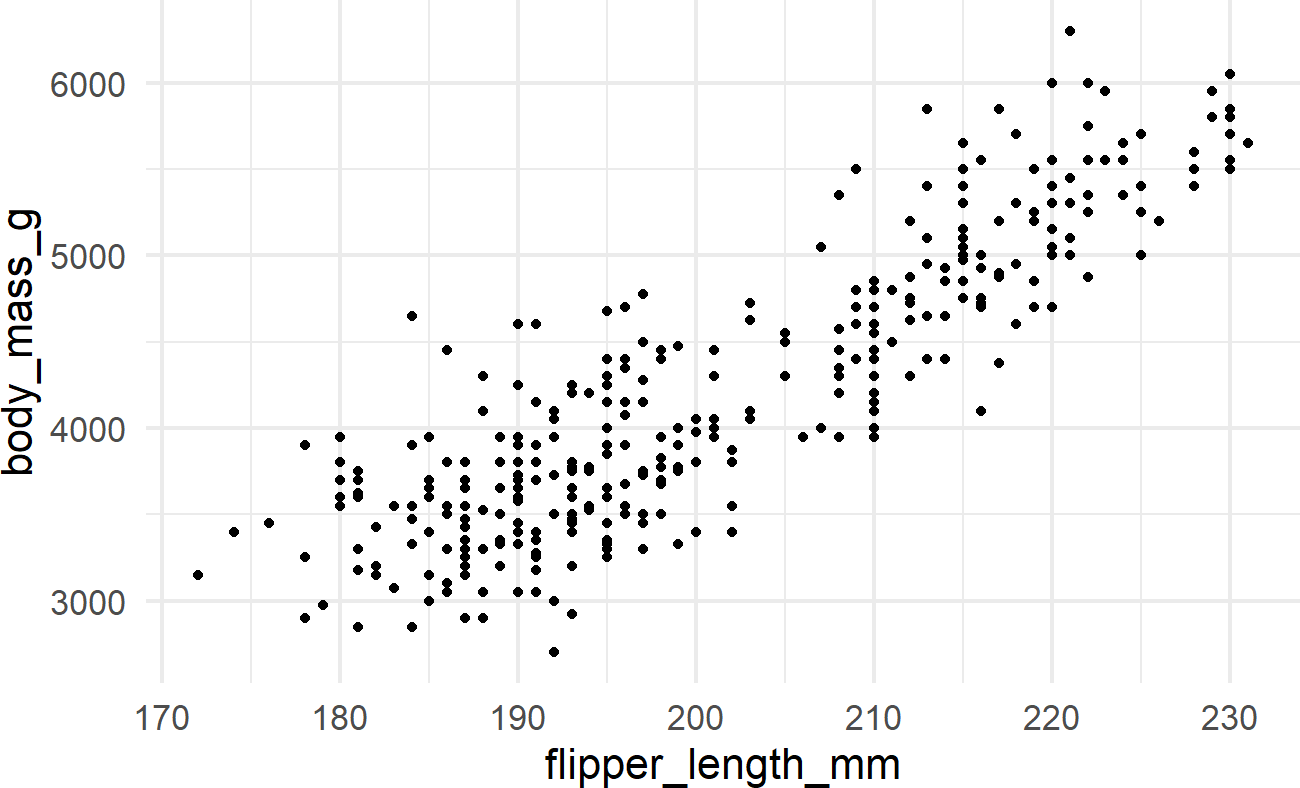### 8.2.3 Other Geoms

We will see a few more geom’s throughout the book, most prominently featured will be geom_col(), geom_density(), geom_histogram(), and geom_linerange(). Each of these has a minimum set of aesthetic mappings which must be specified in order to produce a plot:

geom Required Aesthetics Notes
geom_col() x,y Map x to a discrete variable and y to a continuous variable
geom_density() x Map x to a continuous variable
geom_histogram() x Map x to a contiunuous variable and bin similar x values together
geom_linerange() x,ymin,ymax Map x to a discrete variable and ymin,ymax to two related continuous variables

To show small examples of these other plot types, the following subset of data from the built-in mpg dataset will be used:

## create mpgDF data frame
mpgDF = mpg %>%
group_by(manufacturer) %>%
summarize(cityMPG = mean(cty),
hwyMPG = mean(hwy),
numCarModels = n_distinct(model),
) %>%
filter(numCarModels >= 2)

mpgDF ## view contents of data frame
## # A tibble: 9 x 4
##   manufacturer cityMPG hwyMPG numCarModels
##   <chr>          <dbl>  <dbl>        <int>
## 1 audi            17.6   26.4            3
## 2 chevrolet       15     21.9            4
## 3 dodge           13.1   17.9            4
## 4 ford            14     19.4            4
## 5 hyundai         18.6   26.9            2
## 6 nissan          18.1   24.6            3
## 7 subaru          19.3   25.6            2
## 8 toyota          18.5   24.9            6
## 9 volkswagen      20.9   29.2            4

Small examples are shown below to expose the reader to these capabilities.

#### 8.2.3.1 geom_col

Recall from the dplyr chapter that the chaining operator, %>% makes the object to its left the first argument of the function to its right. Since the first argument to the ggplot function is assumed to be the data argument (see https://ggplot2.tidyverse.org/reference/ggplot.html), mpgDF %>% ggplot() passes the mpgDF data frame as the data argument value used in the ggplot() function; ggplot(mpgDF) and ggplot(data = mpgDF) are other equivalent ways call the function.

mpgDF %>% ## use mpgDF as data argument to ggplot()
ggplot() +
geom_col(aes(x = manufacturer, y = numCarModels))#### 8.2.3.2 geom_density

See https://serialmentor.com/dataviz/histograms-density-plots.html for more information on both density plots and histograms.

mpgDF %>% ## use mpgDF as data argument to ggplot()
ggplot() +
geom_density(aes(x = cityMPG))#### 8.2.3.3 geom_histogram

mpgDF %>% ## use mpgDF as data argument to ggplot()
ggplot() +
geom_histogram(aes(x = cityMPG))#### 8.2.3.4 geom_linerange

mpgDF %>% ## use mpgDF as data argument to ggplot()
ggplot() +
geom_linerange(aes(x = manufacturer,
ymin = cityMPG,
ymax = hwyMPG))A useful trick is to flip the axes when mapping a position aesthetic to long labels such as car manufacturer names:

mpgDF %>% ## use mpgDF as data argument to ggplot()
ggplot() + #change aesthetics to y, xmin, xmax
geom_linerange(aes(y = manufacturer,
xmin = cityMPG,
xmax = hwyMPG))### 8.2.4 Other Elements of the Grammar

Above, we learned that specifying a complete plot required a dataset, a geom, and a minimal set of mappings. Behind the scenes, other grammatical elements were chosen by default; in reality, you can make all of these other decisions explicit. Some of these other elements included a coordinate system, a statistical transformation, and scales:

• Coordinate Systems A coordinate system (coord for short) determines how data coordinates are mapped to the plane of a graphic. The default coordinate system is a two-axis system, think x- and y-coordinates, called the cartesian coordinate system.
• Statistical Transformations: A statistical transformation is a way of manipulating or transforming data prior to its display. It usually is used to summarize data in a meaningful way such as when creating a histogram of one variable or summarizing the relationship of two variables using a linear regression line. These transformations are optional, but can prove useful as shortcuts to get from data to useful visuals.
• Scales: Whereas aesthetic mappings relate data to attributes that you can visually perceive (e.g. color, symbol shapes, fill, etc.), scales dictate how the mapping from data to attribute is performed. For example, a scale might determine which colors are mapped to which values in the data.

We will learn more about these other elements on an as needed basis. For now, we recognize the grammar for what it is, namely a strong foundation for understanding and describing a wide range of graphics.

Go to top of page: link to the top